1. 翻译法（推断法）
2. 百度法（检索法）
3. 打印测试法（核心🌟）
4. 代码拆解法（核心🌟）
5. 进入类里观察法
6. B站视频法

# 2.🌟一个例子，把方法说清楚

``````import d2l
import torch

n_train, n_test, num_inputs, batch_size = 20, 100, 200, 5
true_w, true_b = torch.ones((num_inputs, 1)) * 0.01, 0.05
train_data = d2l.synthetic_data(true_w, true_b, n_train)
test_data = d2l.synthetic_data(true_w, true_b, n_test)

``````

## 第一步：代码来了，啥也不懂就先运行试试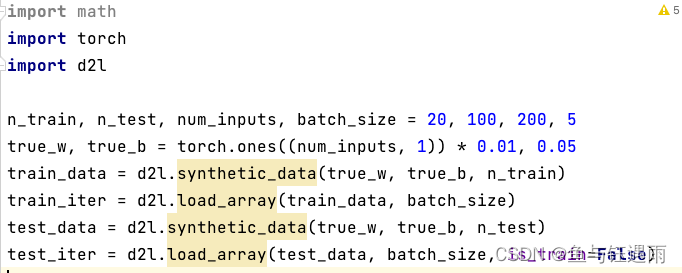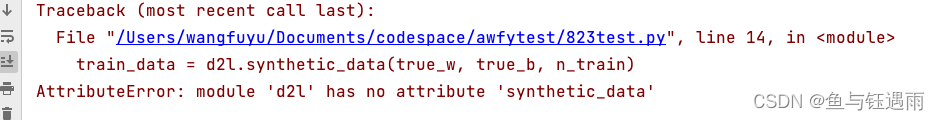### 方法2:遇到报错“复制后百度”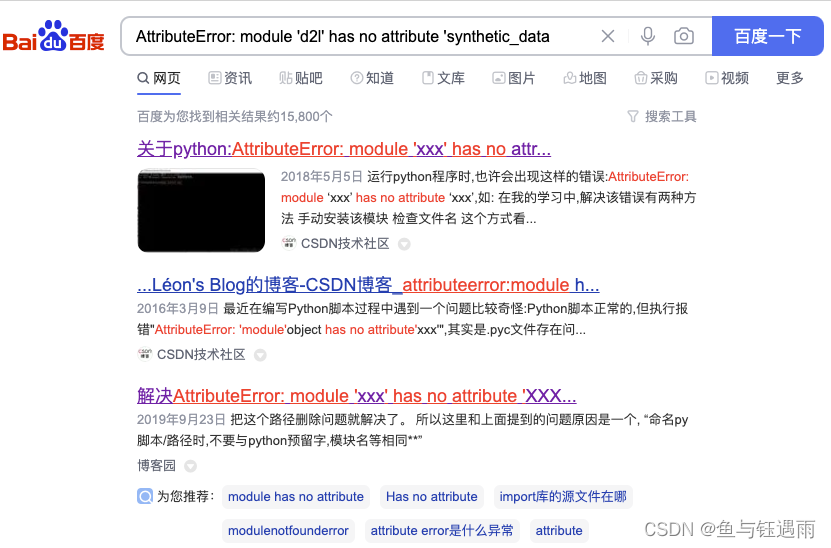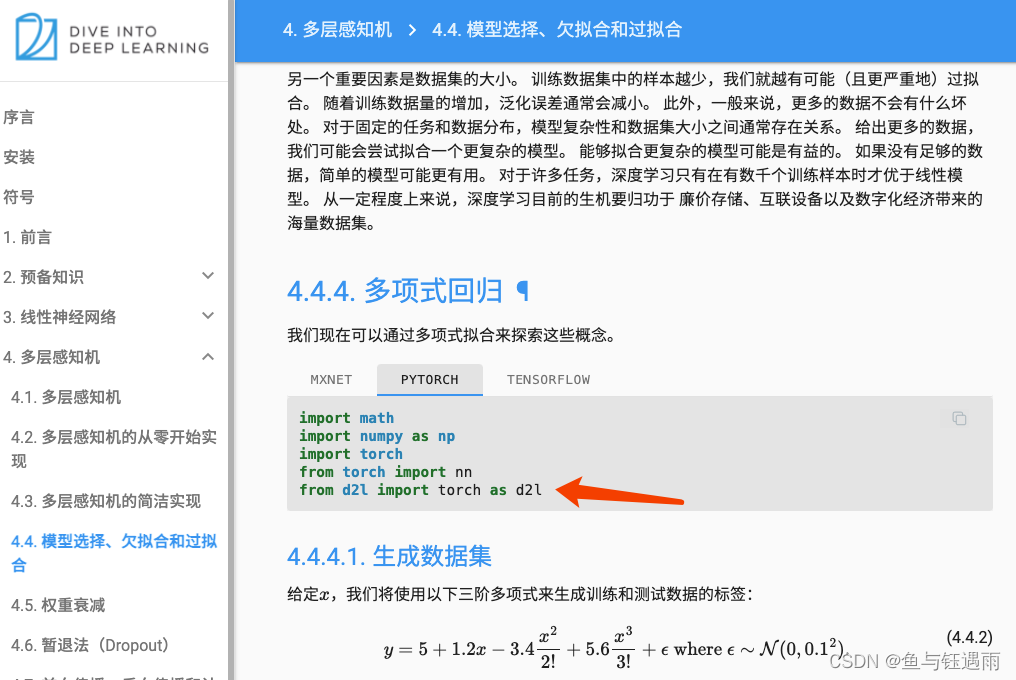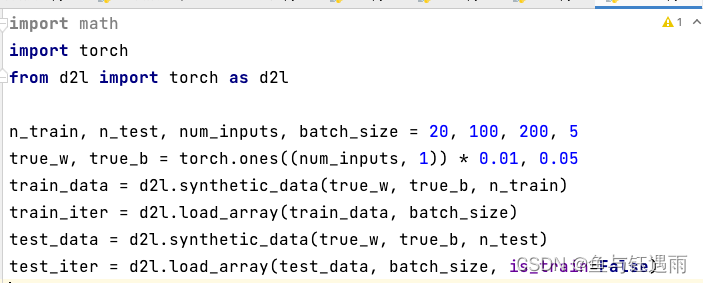## 第二步：跑通代码后，逐句写注释

``````import math #这一句导入math这个包
import torch # 这一句导入torch包
from d2l import torch as d2l #这一句导入d2l中的torch包，并命名为d2l

# 这一句看不懂了？啥意思啊，几个数，几个点？
n_train, n_test, num_inputs, batch_size = 20, 100, 200, 5
``````

### 方法3:通过打印就知道在干什么了！！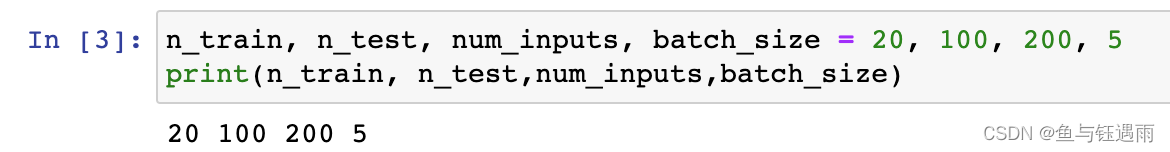`print()是一个`是一个打印方法，可以帮助我们查看变量，从此我们就学会了，如何查看变量了，就是打印，用jupyter notebook运行后打印，这是一个很重要的代码学习方法，一定要记住，当你不知道代码干了什么的时候，你就打印出来过看，然后你就学会了！！！！

### 方法4:代码拆解观察，逐步看懂整个句子

``````import math #这一句导入math这个包
import torch # 这一句导入torch包
from d2l import torch as d2l #这一句导入d2l中的torch包，并命名为d2l

# 这一句代表，为四个变量赋值四个数字，含义就是英语单词的含义
n_train, n_test, num_inputs, batch_size = 20, 100, 200, 5

#这一句又看不懂了！！什么乱七八糟的啊？ torch.ones()是什么？torch.ones(())是什么？
#torch.ones((num_inputs,1))是什么？torch.ones((num_inputs,1))*0.01是什么？
#到底这都是什么啊？ 深度学习好难啊！！！！ 要疯掉了！！！
#啥也不会怎么学啊？？？？？
true_w, true_b = torch.ones((num_inputs, 1)) * 0.01, 0.05
``````

1. 首先，通过提问题的方式对代码进行拆解，上面已经通过大量的疑问句把这个代码拆解成了很多小段了？
2. 拆解完成之后就是利用上面讲过的方法2：百度和方法3：打印观察来分析每一个小东西是什么，最后弄明白整行代码！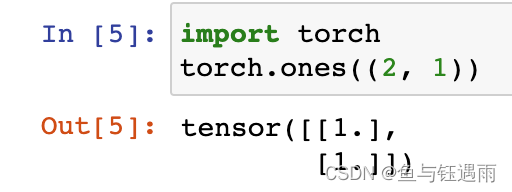`torch.ones((num_inputs, 1)) * 0.01`是什么意思呢？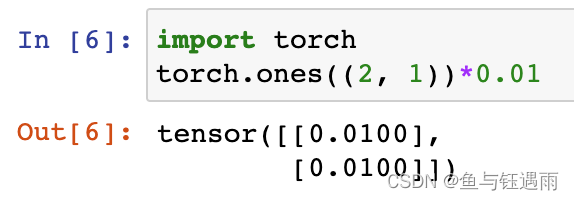`true_w, true_b = torch.ones((num_inputs, 1)) * 0.01, 0.05`这一整句话是什么意思呢？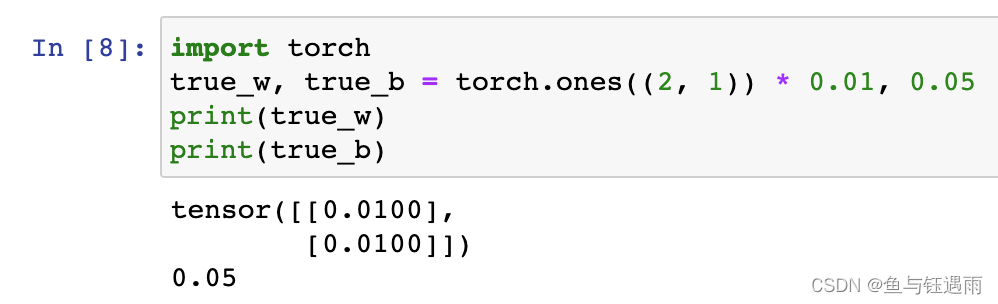### 方法5:进入类里，逐句看方法

``````import math #这一句导入math这个包
import torch # 这一句导入torch包
from d2l import torch as d2l #这一句导入d2l中的torch包，并命名为d2l

# 这一句代表，为四个变量赋值四个数字，含义就是英语单词的含义
n_train, n_test, num_inputs, batch_size = 20, 100, 200, 5

#生成矩阵true_w和数字b
true_w, true_b = torch.ones((num_inputs, 1)) * 0.01, 0.05
# 这一句又看不懂了，啥啊，synthetic_data到底是在干什么？ 是什么啊？
# 真的无语了？哪里来的synthetic_data啊？
train_data = d2l.synthetic_data(true_w, true_b, n_train)
``````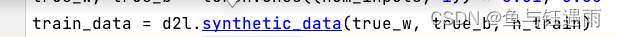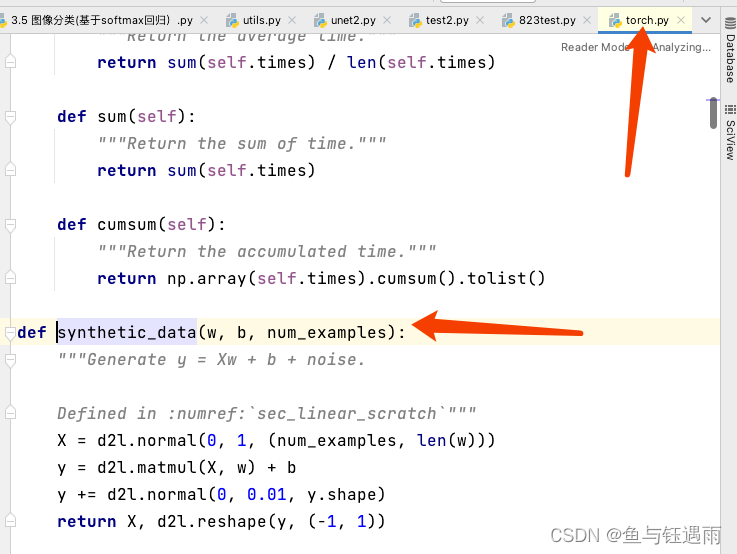``````# 一个方法，输入了3个变量，分别是w,b,num_examples
def synthetic_data(w, b, num_examples):
# 对方法的解释，翻译为：产生 y= Xw+ b + noise
"""Generate y = Xw + b + noise.
Defined in :numref:`sec_linear_scratch`"""
# 这句看不懂
X = d2l.normal(0, 1, (num_examples, len(w)))
# 这句看不懂
y = d2l.matmul(X, w) + b
# 这句看不懂
y += d2l.normal(0, 0.01, y.shape)
# 这个是返回了X，d2l.reshape(y,(-1,1))
return X, d2l.reshape(y, (-1, 1))
``````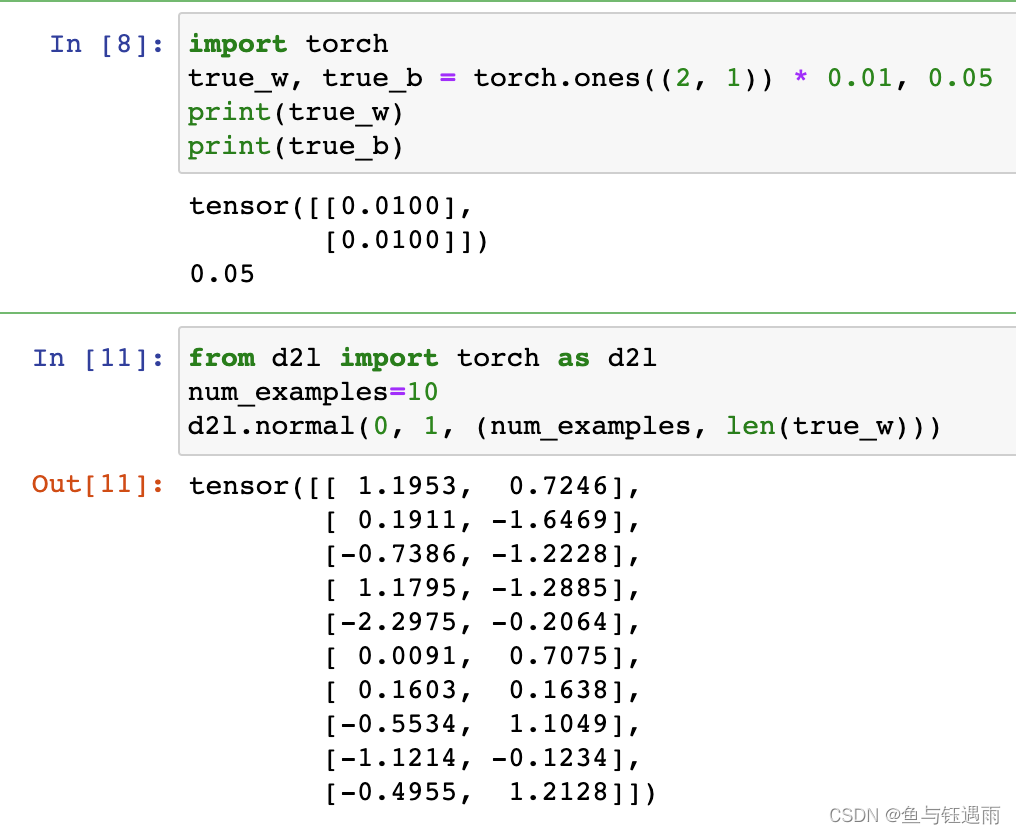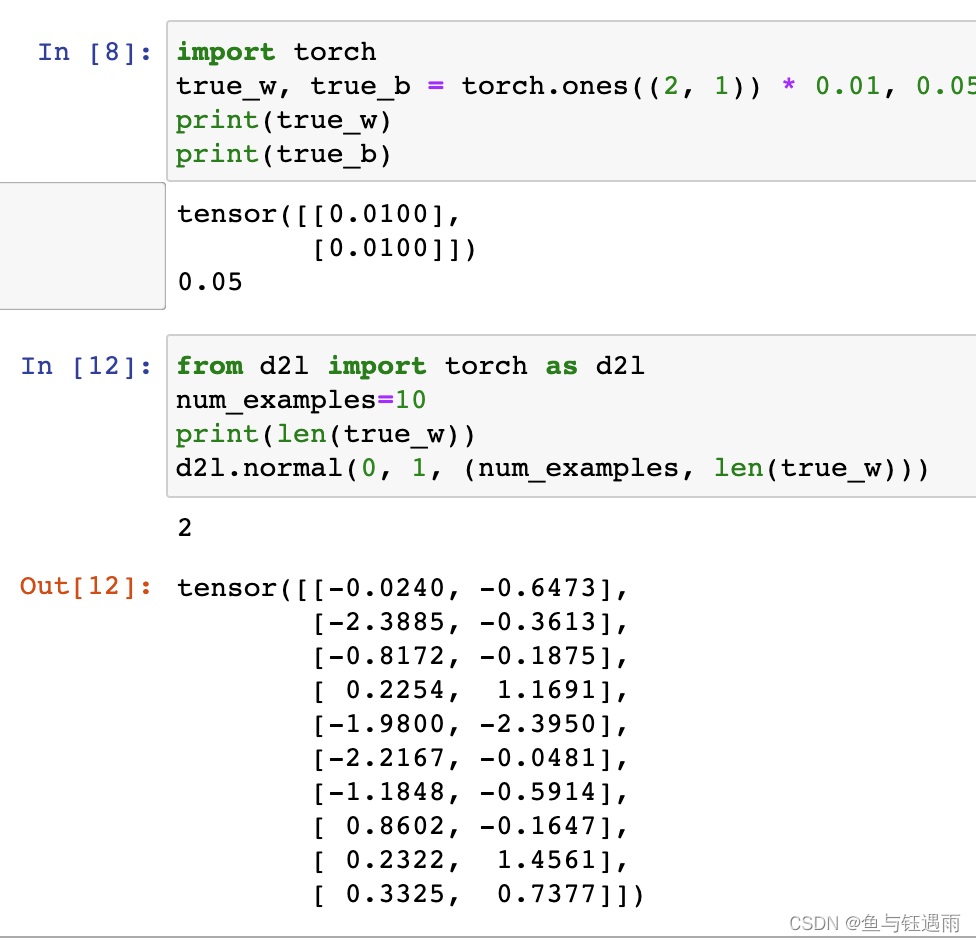``````# 一个方法，输入了3个变量，分别是w,b,num_examples
def synthetic_data(w, b, num_examples):
# 对方法的解释，翻译为：产生 y= Xw+ b + noise
"""Generate y = Xw + b + noise.
Defined in :numref:`sec_linear_scratch`"""
# 生成尺寸为(num_examples, len(w))的随机矩阵
X = d2l.normal(0, 1, (num_examples, len(w)))
# 这句看不懂？ 测试法:矩阵乘法+常数b
y = d2l.matmul(X, w) + b
# 这句看不懂？ 测试法：生成均值为0，方差为0.01的和y尺寸相同的随机数矩阵，然后加到y上，其实就是实现了y= Xw+ b + noise
y += d2l.normal(0, 0.01, y.shape)
# 这个是返回了X，d2l.reshape(y,(-1,1))
# d2l.reshape(y,(-1,1))是什么意思呢？ 测试法！！！
# 通过测试可知，返回num_examples*len(w)尺寸的特征矩阵和num_examples*1的标签矩阵
return X, d2l.reshape(y, (-1, 1))
``````

y = d2l.matmul(X, w) + b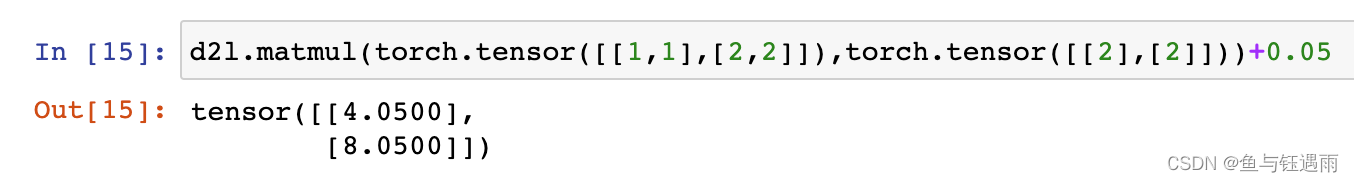y += d2l.normal(0, 0.01, y.shape)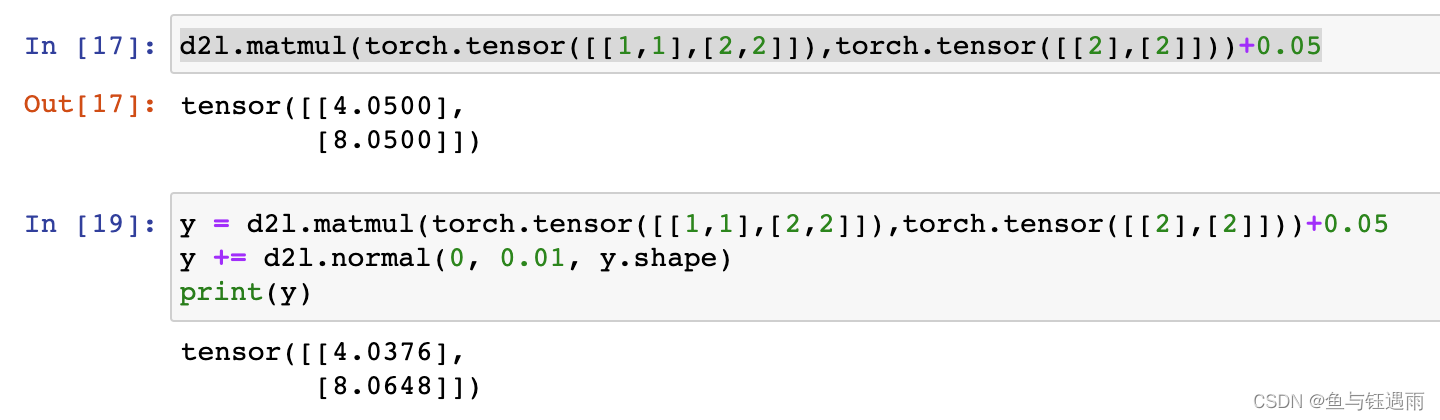d2l.reshape(y, (-1, 1))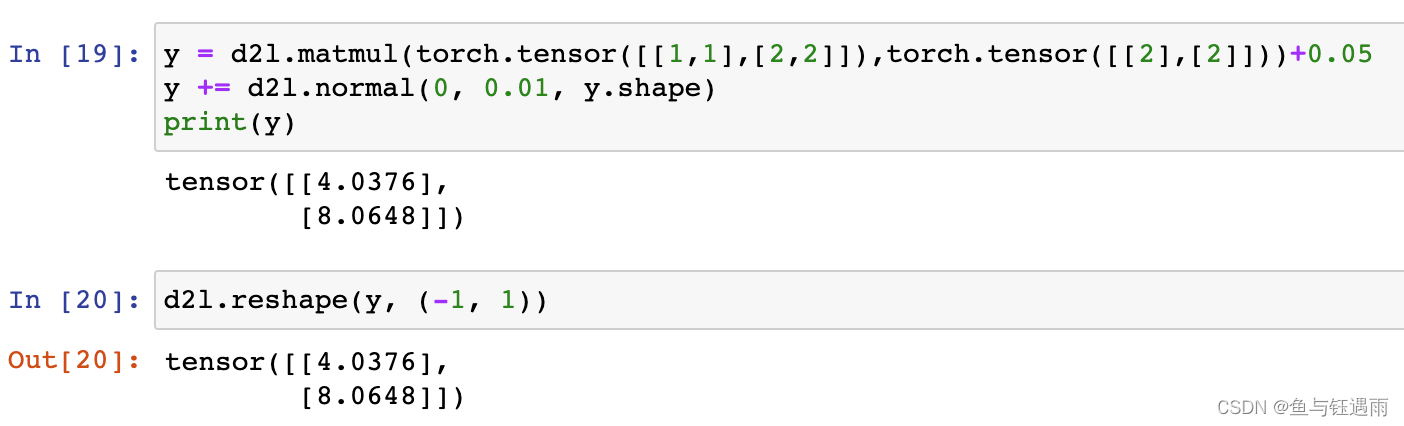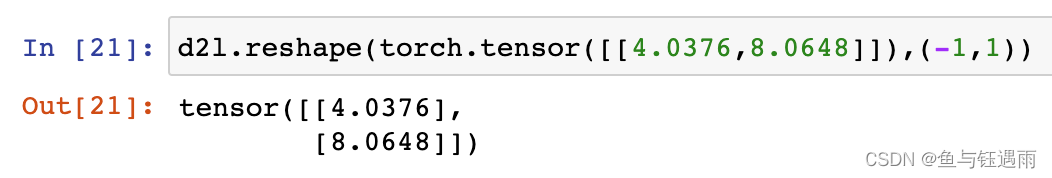### 方法6:B站视频法

https://www.bilibili.com/

# 3.总结

``````train_iter = d2l.load_array(train_data, batch_size)
test_data = d2l.synthetic_data(true_w, true_b, n_test)
``````

## 6. B站视频法